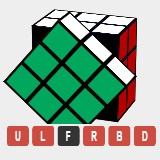# Introduction to the Rubik's Cube – Notation, Algorithms and moreFor the first lesson, no part of the solving method will be covered. This is to give students time to be able to familiarize with the cube a bit before jumping straight into learning it. This is especially helpful especially to students who haven't tried to solve a Rubik's Cube before, but it also helps all students gather an understanding of the cube itself and common vocabulary that will be used throughout the curriculum.

Make sure that cubes are distributed evenly and they are solved. Do not let the students scramble their cubes, as the solved cubes will be used later on.

## IntroductionAt the beginning of the presentation, the students will be introduced to the Rubik's Cube with some information given on its history and the popularity of the puzzle today. Following this, the end goals for the curriculum will be provided, explaining to students what they will be able to achieve at the end and giving them targets to aim for using a Bronze -> Diamond structure (this can be accompanied with certificates following the completion of the course). The method that will be used is named and its potential is noted, however no overview of the method is given yet as to not confuse the students.

## Algorithms

The next few slides outline the use of algorithms in a Rubik's Cube solve. The differences between algorithmic and intuitive stages are explained, without going into detail about the actual stages of the solve just yet. The definition of an algorithm is also given, as algorithms are used in other fields such as computing in a similar way. The students may be worried about the idea of an intuitive stage where they have no help and just have to turn the cube, however the second slide explains how the intuitive part of the basic method that will be used is nothing to worry about. Make sure that this is stressed, as students may be put off by the idea without even picking up a cube.

## Notation

The standard Rubik's Cube notationis explained in the next slides, and the differences between the different types of turns are described. Images are used to show all three types of move. This could also be mimicked with the physical cubes for a better understanding. Knowing whether or not a turn you make is clockwise or counter-clockwise may confuse some students, so the next slide explains how similar turns can be confused and warns students to take care when performing them.

Scrambles (using standard notation) are also explained, and an example is given. On the next few slides is an interactive scramble, in which the students get to try scrambling the cubes they have using a scramble given. A net of the correctly scrambled cube is provided which can be used to check. If the students haven't been able to scramble the cube correctly then don't worry, as no specific scramble will be used to learn the solution later on. Try the online Rubik's Cube scramble generator, which is used on official competitions to assure equal to competitors.

The lesson is then closed by explaining the origin of the scramble they just attempted to perform, which is used to highlight the power of the skill they are about to learn.

#### Rubik’s Cube Curriculum SeriesDownload The PowerPoint Presentations from Google Drive